Courses

# Retro (Past 13 Years) IIT - JEE Advanced (Thermodynamics)

## 14 Questions MCQ Test Chemistry for JEE Advanced | Retro (Past 13 Years) IIT - JEE Advanced (Thermodynamics)

Description
This mock test of Retro (Past 13 Years) IIT - JEE Advanced (Thermodynamics) for JEE helps you for every JEE entrance exam. This contains 14 Multiple Choice Questions for JEE Retro (Past 13 Years) IIT - JEE Advanced (Thermodynamics) (mcq) to study with solutions a complete question bank. The solved questions answers in this Retro (Past 13 Years) IIT - JEE Advanced (Thermodynamics) quiz give you a good mix of easy questions and tough questions. JEE students definitely take this Retro (Past 13 Years) IIT - JEE Advanced (Thermodynamics) exercise for a better result in the exam. You can find other Retro (Past 13 Years) IIT - JEE Advanced (Thermodynamics) extra questions, long questions & short questions for JEE on EduRev as well by searching above.
QUESTION: 1

Solution:
QUESTION: 2

Solution:
QUESTION: 3

### For the process,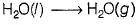at T = 100° C and 1 atm pressure, the correct choice is (2014 Adv., Single Option Correct)

Solution:
QUESTION: 4

The standard enthalpies of for mation of CO2(g), H2O (l) and glucose (s) at 25°C are - 400 kJ / mol, - 300 kJ / mol and - 1300 kJ / mol, respectively .

The standard enthalpy of combustion per gram Of glucose at 25°C is

Solution:
QUESTION: 5

Using the data provided, calculate the multiple bond energy (kJ mol-1) of a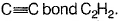That energy is (take the bond energy of a C—H bond as 350 kJ mol -1)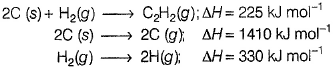(2012 , Single Option Correct)

Solution:
QUESTION: 6

Match the trans formation in Column I with appropriate options in Column II.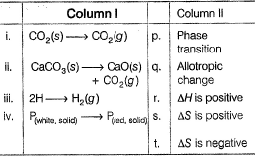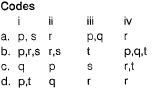Solution:
*Answer can only contain numeric values
QUESTION: 7

One mole of an ideal gas is taken from a to b along two paths denoted by the solid and the dashed lines as shown in the graph below, If the work done along the solid line path is Ws and that along the dotted line path is Wd , then the integer closest to the ratio W/ Ws is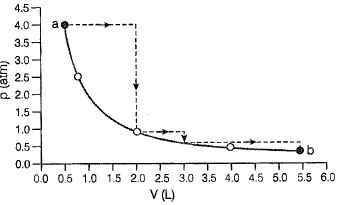Solution:
QUESTION: 8

Direction (Q. Nos. 8-9) These questions are based on Statement I and Statement II. Select the correct answer from the codes given below.

Q.

Statement I : For every chemical reaction at equilibrium , standard Gibbs ’ energy of reaction is zero.

Statement II : At constant temperature and pressure, chemical reactions are spontaneous in the direction of decreasing Gibbs ’ energy .

Solution:
QUESTION: 9

Satement I : There is a natural asymmetry between converting work to heat and converting heat to work.

Statement II : No process is possible in which the sole result is the absorption of heat from a reservoir and its complete conversion into work.

(2008, Statement Type)

Solution:
QUESTION: 10

For the process H2O (l) (1 bar, 373 K) → H2O (g) (1 bar, 373 K), the correct set of thermodynamic parameters is

(2007, Single Option Correct)

Solution:
QUESTION: 11

The value of log10 K for a reaction,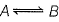is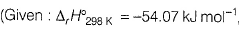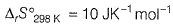and
R = 8.314 JK-1 mol-1; 2 .303 x 8.314 x 298 = 5705)

(2007, Single Option Correct)

Solution:
QUESTION: 12

The direct conversion of A to B is difficult, hence it is carried out by the following shown path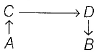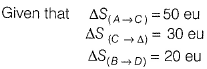where eu is entropy unit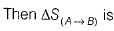(2006, Single Option Correct)

Solution:
QUESTION: 13

A monatomic ideal gas undergoes a process in which the ratio of p to V at any instant is constant and equals to 1. What is the molar heat capacity of the gas ?

Solution:
SO3 ⇒ 3 bond pairs ⇒ sp2 hybrid ⇒ trigonal planar
BrF3 ⇒ 3 bond pairs, 2 lone pairs ⇒ sp3d hybrid ⇒ T-shaped
(SiO3)2- ⇒ 3 bond pairs ⇒ sp2 ⇒ trig. plan.
OSF2 ⇒ 3 b.p., 1 l.p. ⇒ sp3 hybrid ⇒ pyramidal.

QUESTION: 14

One mole of monatomic ideal gas expands adiabatically at initial temperature T against a constant external pressure of 1 atm from 1 L to 2 L. Find out the final temperature (R = 0.0821 Latm K-1 mol-1)

(2005, Single Option Correct)

Solution: